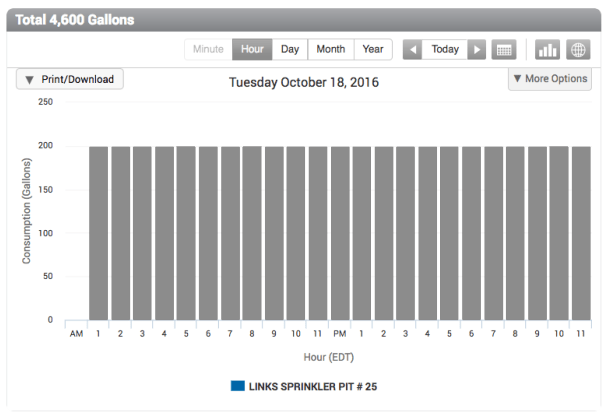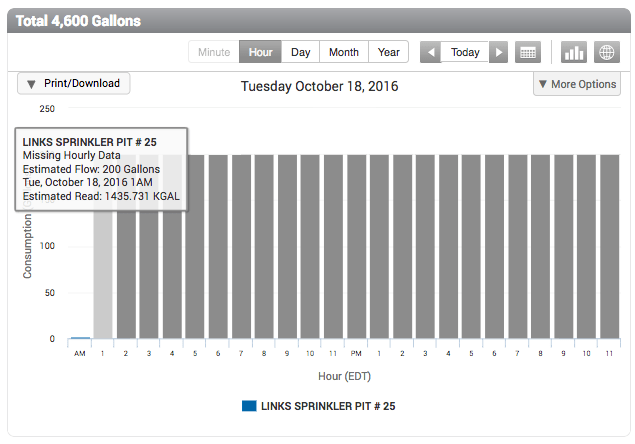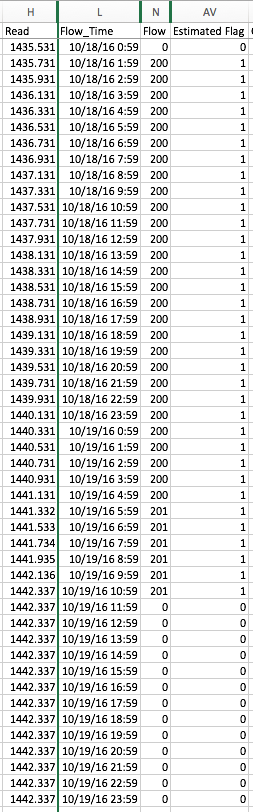# Estimated Reads, Endpoints and Data Backfill

Q: What happens when communications between an endpoint and BEACON is interrupted? Will missing reads be backfilled when communication is re-established? When does BEACON estimate reads?
A:
“Backfill” is the process of filling in missing data, typically meter reads that were not received because a scheduled communication with an endpoint was missed. When communication is re-established and the next endpoint message includes the missing reads, those reads will be “backfilled” and shown as blue bars in the Monitor page Consumption Graph.

In the unlikely event there is a gap that has not been backfilled by the data received from the endpoint, BEACON shows an aggregate read (also known as an estimated read) over the time period by calculating the total amount of consumption that occurred during that period and evenly distributing it across the gap. The aggregate read is then displayed as equal gray bars. This produces Consumption Graphs that look like the one below.

The process assures that reads will be available for the billing.

NOTE: Estimated reads for ORION Cellular endpoint data cannot be “backfilled” with actual reads.Gray bars tell when consumption data is an estimate. To get more details:

1. Hover the cursor over a gray bar in the Monitor page consumption graph. The tooltip shows the total estimated flow that was calculated as an average from missing hourly data.2. Use the Actions drop-down menu to, for example, Export Data>Date Range and choose a start date that comes before the date displayed in the Consumption Graph. Then open the file in Excel to see if an estimated flag was set by looking at the Estimated Flag column (column AV in the example below).

In addition you can look at columns:

• L to see when flow was reported by the endpoint
• N to see the flow total.BEACON calculated the total flow measured between the valid read of 1435.531 at 0:59 on the 18th and the valid read of 1442.337 at 11:59 on the 19th. It then evenly distributed the result of 6.806 KGallons (the unit of measure in this example) as estimated reads across the 34 hours between valid reads.The data in this example is the same data that generated the Consumption Graph for LINKS SPRINKLER PIT #25 (above).

• There are times when estimated reads get distributed unevenly, as shown in the Consumption Graph below.This uneven distribution is the result of meter resolution, which in this example is 10. That is, each revolution of the meter’s disc measures 10 gallons. As a result, BEACON distributes estimated reads for this particular meter in 10-gallon increments. Between 12 AM and 6 PM, a total of 250 gallons was used, which BEACON distributed as 10 + 20 + 10 + 10 + 20 + 10 + 10 + 10 + 20 + 10 + 10 + 20 + 10 + 10 + 10 + 20+ 10 + 10 + 20 = 250.